Anzeige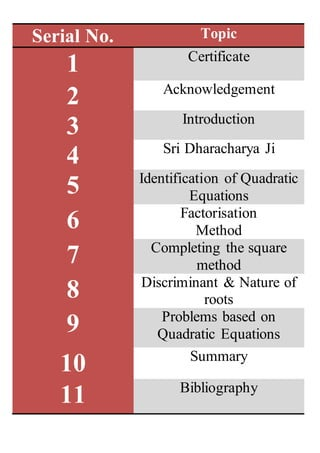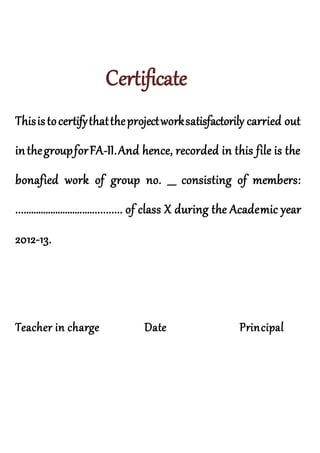Anzeige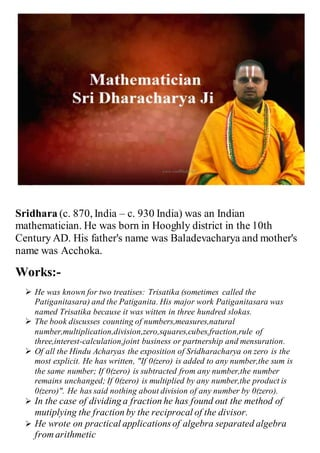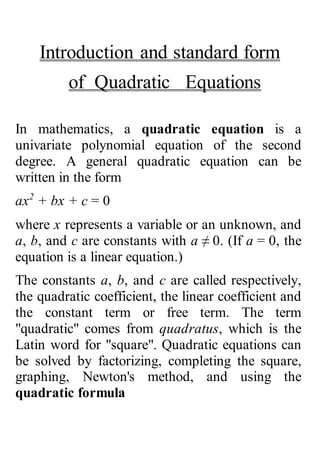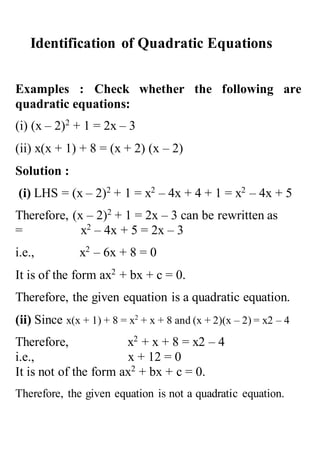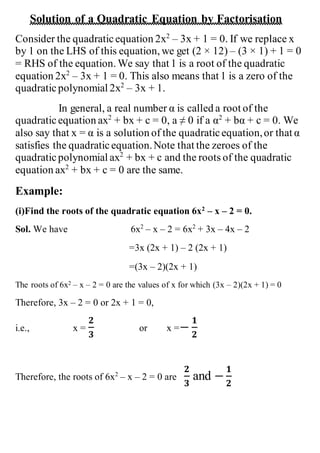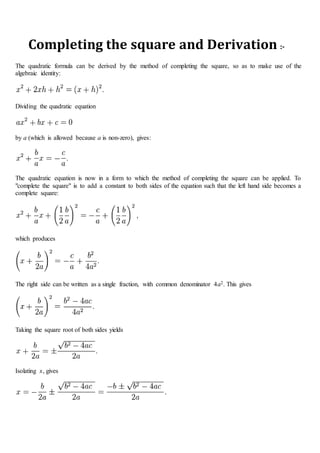Anzeige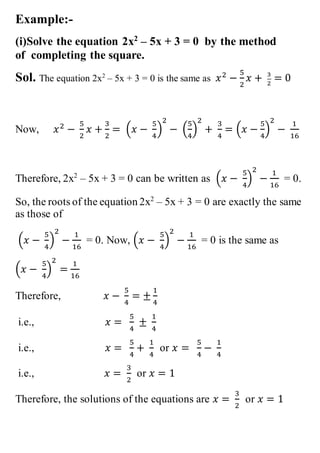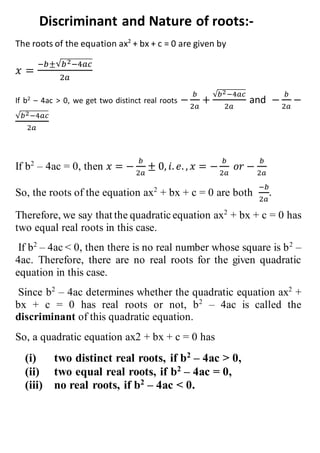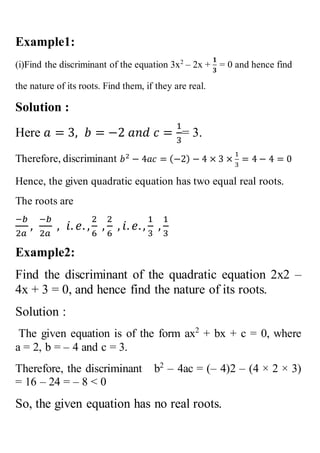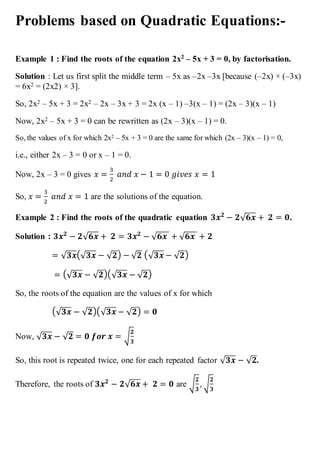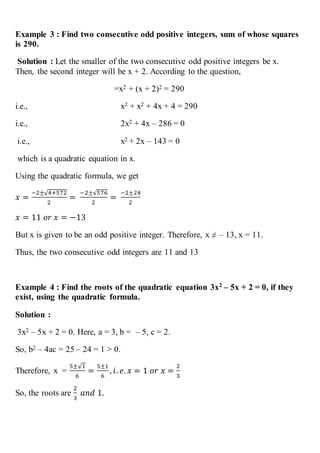Anzeige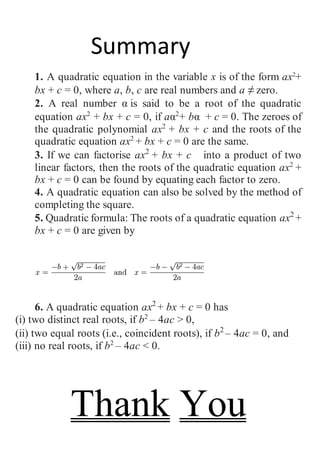Nächste SlideShare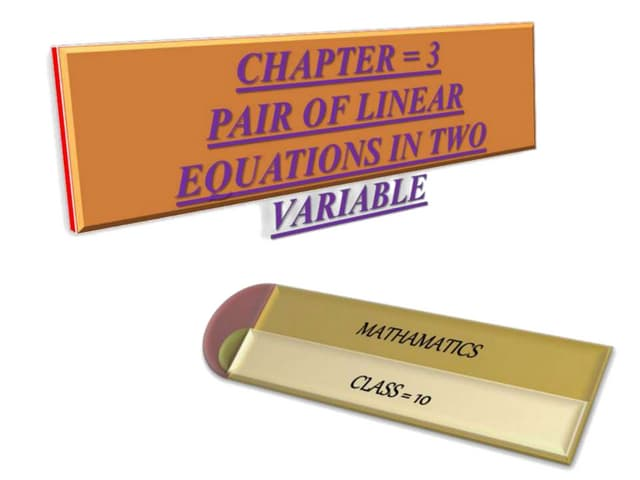PROJECT (PPT) ON PAIR OF LINEAR EQUATIONS IN TWO VARIABLES - CLASS 10
1 von 16
Anzeige

2. Serial No. Topic 1 Certificate 2 Acknowledgement 3 Introduction 4 Sri Dharacharya Ji 5 Identification of Quadratic Equations 6 Factorisation Method 7 Completing the square method 8 Discriminant & Nature of roots 9 Problems based on Quadratic Equations 10 Summary 11 Bibliography
3. Certificate Thisistocertifythattheprojectworksatisfactorily carried out inthegroupforFA-II.And hence, recorded in this file is the bonafied work of group no. ___ consisting of members: .......................................... of class X during the Academic year 2012-13. Teacher in charge Date Principal
4. Acknowledgement SpecialthankstoMrs.................madam for her guidance and hersupport.Shehelpedthroughouttheproject.We thank our Principalforhersupport. We thank our group members for theircontribution and cooperation for making this project. ThisProjectwasmadefrom the support and contribution of our group members. So we will thank each one of us.
5. Sridhara (c. 870, India – c. 930 India) was an Indian mathematician. He was born in Hooghly district in the 10th Century AD. His father's name was Baladevacharya and mother's name was Acchoka. Works:-  He was known for two treatises: Trisatika (sometimes called the Patiganitasara) and the Patiganita. His major work Patiganitasara was named Trisatika because it was witten in three hundred slokas.  The book discusses counting of numbers,measures,natural number,multiplication,division,zero,squares,cubes,fraction,rule of three,interest-calculation,joint business or partnership and mensuration.  Of all the Hindu Acharyas the exposition of Sridharacharya on zero is the most explicit. He has written, "If 0(zero) is added to any number,the sum is the same number; If 0(zero) is subtracted from any number,the number remains unchanged; If 0(zero) is multiplied by any number,the product is 0(zero)". He has said nothing about division of any number by 0(zero).  In the case of dividing a fraction he has found out the method of mutiplying the fraction by the reciprocal of the divisor.  He wrote on practical applicationsof algebra separated algebra from arithmetic
6. Introduction and standard form of Quadratic Equations In mathematics, a quadratic equation is a univariate polynomial equation of the second degree. A general quadratic equation can be written in the form ax2 + bx + c = 0 where x represents a variable or an unknown, and a, b, and c are constants with a ≠ 0. (If a = 0, the equation is a linear equation.) The constants a, b, and c are called respectively, the quadratic coefficient, the linear coefficient and the constant term or free term. The term "quadratic" comes from quadratus, which is the Latin word for "square". Quadratic equations can be solved by factorizing, completing the square, graphing, Newton's method, and using the quadratic formula
7. Identification of Quadratic Equations Examples : Check whether the following are quadratic equations: (i) (x – 2)2 + 1 = 2x – 3 (ii) x(x + 1) + 8 = (x + 2) (x – 2) Solution : (i) LHS = (x – 2)2 + 1 = x2 – 4x + 4 + 1 = x2 – 4x + 5 Therefore, (x – 2)2 + 1 = 2x – 3 can be rewritten as = x2 – 4x + 5 = 2x – 3 i.e., x2 – 6x + 8 = 0 It is of the form ax2 + bx + c = 0. Therefore, the given equation is a quadratic equation. (ii) Since x(x + 1) + 8 = x2 + x + 8 and (x + 2)(x – 2) = x2 – 4 Therefore, x2 + x + 8 = x2 – 4 i.e., x + 12 = 0 It is not of the form ax2 + bx + c = 0. Therefore, the given equation is not a quadratic equation.
8. Solution of a Quadratic Equation by Factorisation Consider the quadraticequation 2x2 – 3x + 1 = 0. If we replace x by 1 on the LHS of this equation, we get (2 × 12) – (3 × 1) + 1 = 0 = RHS of the equation. We say that 1 is a root of the quadratic equation 2x2 – 3x + 1 = 0. This also means that 1 is a zero of the quadraticpolynomial 2x2 – 3x + 1. In general, a real number α is called a root of the quadraticequation ax2 + bx + c = 0, a ≠ 0 if a α2 + bα + c = 0. We also say that x = α is a solution of the quadraticequation,or that α satisfies the quadraticequation.Note that the zeroes of the quadraticpolynomial ax2 + bx + c and the roots of the quadratic equation ax2 + bx + c = 0 are the same. Example: (i)Find the roots of the quadratic equation 6x2 – x – 2 = 0. Sol. We have 6x2 – x – 2 = 6x2 + 3x – 4x – 2 =3x (2x + 1) – 2 (2x + 1) =(3x – 2)(2x + 1) The roots of 6x2 – x – 2 = 0 are the values of x for which (3x – 2)(2x + 1) = 0 Therefore, 3x – 2 = 0 or 2x + 1 = 0, i.e., x = 𝟐 𝟑 or x =− 𝟏 𝟐 Therefore, the roots of 6x2 – x – 2 = 0 are 𝟐 𝟑 and − 𝟏 𝟐
9. Completing the square and Derivation :- The quadratic formula can be derived by the method of completing the square, so as to make use of the algebraic identity: Dividing the quadratic equation by a (which is allowed because a is non-zero), gives: The quadratic equation is now in a form to which the method of completing the square can be applied. To "complete the square" is to add a constant to both sides of the equation such that the left hand side becomes a complete square: which produces The right side can be written as a single fraction, with common denominator 4a2. This gives Taking the square root of both sides yields Isolating x, gives
10. Example:- (i)Solve the equation 2x2 – 5x + 3 = 0 by the method of completing the square. Sol. The equation 2x2 – 5x + 3 = 0 is the same as 𝑥2 − 5 2 𝑥 + 3 2 = 0 Now, 𝑥2 − 5 2 𝑥 + 3 2 = (𝑥 − 5 4 ) 2 − ( 5 4 ) 2 + 3 4 = (𝑥 − 5 4 ) 2 − 1 16 Therefore, 2x2 – 5x + 3 = 0 can be written as (𝑥 − 5 4 ) 2 − 1 16 = 0. So, the roots of the equation 2x2 – 5x + 3 = 0 are exactly the same as those of (𝑥 − 5 4 ) 2 − 1 16 = 0. Now, (𝑥 − 5 4 ) 2 − 1 16 = 0 is the same as (𝑥 − 5 4 ) 2 = 1 16 Therefore, 𝑥 − 5 4 = ± 1 4 i.e., 𝑥 = 5 4 ± 1 4 i.e., 𝑥 = 5 4 + 1 4 or 𝑥 = 5 4 − 1 4 i.e., 𝑥 = 3 2 or 𝑥 = 1 Therefore, the solutions of the equations are 𝑥 = 3 2 or 𝑥 = 1
11. Discriminant and Nature of roots:- The roots of the equation ax2 + bx + c = 0 are given by 𝑥 = −𝑏±√ 𝑏2−4𝑎𝑐 2𝑎 If b2 – 4ac > 0, we get two distinct real roots − 𝑏 2𝑎 + √𝑏2−4𝑎𝑐 2𝑎 and − 𝑏 2𝑎 − √𝑏2−4𝑎𝑐 2𝑎 If b2 – 4ac = 0, then 𝑥 = − 𝑏 2𝑎 ± 0, 𝑖. 𝑒. , 𝑥 = − 𝑏 2𝑎 𝑜𝑟 − 𝑏 2𝑎 So, the roots of the equation ax2 + bx + c = 0 are both −𝑏 2𝑎 . Therefore, we say that the quadraticequation ax2 + bx + c = 0 has two equal real roots in this case. If b2 – 4ac < 0, then there is no real number whose square is b2 – 4ac. Therefore, there are no real roots for the given quadratic equation in this case. Since b2 – 4ac determines whether the quadratic equation ax2 + bx + c = 0 has real roots or not, b2 – 4ac is called the discriminant of this quadratic equation. So, a quadratic equation ax2 + bx + c = 0 has (i) two distinct real roots, if b2 – 4ac > 0, (ii) two equal real roots, if b2 – 4ac = 0, (iii) no real roots, if b2 – 4ac < 0.
12. Example1: (i)Find the discriminant of the equation 3x2 – 2x + 𝟏 𝟑 = 0 and hence find the nature of its roots. Find them, if they are real. Solution : Here 𝑎 = 3, 𝑏 = −2 𝑎𝑛𝑑 𝑐 = 1 3 = 3. Therefore, discriminant 𝑏2 − 4𝑎𝑐 = (−2) − 4 × 3 × 1 3 = 4 − 4 = 0 Hence, the given quadratic equation has two equal real roots. The roots are −𝑏 2𝑎 , −𝑏 2𝑎 , 𝑖. 𝑒. , 2 6 , 2 6 , 𝑖. 𝑒., 1 3 , 1 3 Example2: Find the discriminant of the quadratic equation 2x2 – 4x + 3 = 0, and hence find the nature of its roots. Solution : The given equation is of the form ax2 + bx + c = 0, where a = 2, b = – 4 and c = 3. Therefore, the discriminant b2 – 4ac = (– 4)2 – (4 × 2 × 3) = 16 – 24 = – 8 < 0 So, the given equation has no real roots.
13. Problems based on Quadratic Equations:- Example 1 : Find the roots of the equation 2x2 – 5x + 3 = 0, by factorisation. Solution : Let us first split the middle term – 5x as –2x –3x [because (–2x) × (–3x) = 6x2 = (2x2) × 3]. So, 2x2 – 5x + 3 = 2x2 – 2x – 3x + 3 = 2x (x – 1) –3(x – 1) = (2x – 3)(x – 1) Now, 2x2 – 5x + 3 = 0 can be rewritten as (2x – 3)(x – 1) = 0. So, the values of x for which 2x2 – 5x + 3 = 0 are the same for which (2x – 3)(x – 1) = 0, i.e., either 2x – 3 = 0 or x – 1 = 0. Now, 2x – 3 = 0 gives 𝑥 = 3 2 𝑎𝑛𝑑 𝑥 − 1 = 0 𝑔𝑖𝑣𝑒𝑠 𝑥 = 1 So, 𝑥 = 3 2 𝑎𝑛𝑑 𝑥 = 1 are the solutions of the equation. Example 2 : Find the roots of the quadratic equation 𝟑𝒙 𝟐 − 𝟐√ 𝟔𝒙 + 𝟐 = 𝟎. Solution : 𝟑𝒙 𝟐 − 𝟐√ 𝟔𝒙 + 𝟐 = 𝟑𝒙 𝟐 − √ 𝟔𝒙 + √ 𝟔𝒙 + 𝟐 = √ 𝟑𝒙(√ 𝟑𝒙 − √ 𝟐) − √ 𝟐 (√ 𝟑𝒙 − √ 𝟐) = (√ 𝟑𝒙 − √ 𝟐)(√ 𝟑𝒙 − √ 𝟐) So, the roots of the equation are the values of x for which (√ 𝟑𝒙 − √ 𝟐)(√ 𝟑𝒙 − √ 𝟐) = 𝟎 Now, √ 𝟑𝒙 − √ 𝟐 = 𝟎 𝒇𝒐𝒓 𝒙 = √ 𝟐 𝟑 So, this root is repeated twice, one for each repeated factor √ 𝟑𝒙 − √ 𝟐. Therefore, the roots of 𝟑𝒙 𝟐 − 𝟐√ 𝟔𝒙 + 𝟐 = 𝟎 are √ 𝟐 𝟑 , √ 𝟐 𝟑
14. Example 3 : Find two consecutive odd positive integers, sum of whose squares is 290. Solution : Let the smaller of the two consecutive odd positive integers be x. Then, the second integer will be x + 2. According to the question, =x2 + (x + 2)2 = 290 i.e., x2 + x2 + 4x + 4 = 290 i.e., 2x2 + 4x – 286 = 0 i.e., x2 + 2x – 143 = 0 which is a quadratic equation in x. Using the quadratic formula, we get 𝑥 = −2±√4+572 2 = −2±√576 2 = −2±24 2 𝑥 = 11 𝑜𝑟 𝑥 = −13 But x is given to be an odd positive integer. Therefore, x ≠ – 13, x = 11. Thus, the two consecutive odd integers are 11 and 13 Example 4 : Find the roots of the quadratic equation 3x2 – 5x + 2 = 0, if they exist, using the quadratic formula. Solution : 3x2 – 5x + 2 = 0. Here, a = 3, b = – 5, c = 2. So, b2 – 4ac = 25 – 24 = 1 > 0. Therefore, x = 5±√1 6 = 5±1 6 , 𝑖. 𝑒. 𝑥 = 1 𝑜𝑟 𝑥 = 2 3 So, the roots are 2 3 𝑎𝑛𝑑 1.
15. Summary 1. A quadratic equation in the variable x is of the form ax2 + bx + c = 0, where a, b, c are real numbers and a ≠ zero. 2. A real number αis said to be a root of the quadratic equation ax2 + bx + c = 0, if aα + bα+ c = 0. The zeroes of the quadratic polynomial ax2 + bx + c and the roots of the quadratic equation ax2 + bx + c = 0 are the same. 3. If we can factorise ax2 + bx + c into a product of two linear factors, then the roots of the quadratic equation ax2 + bx + c = 0 can be found by equating each factor to zero. 4. A quadratic equation can also be solved by the method of completing the square. 5. Quadratic formula: The roots of a quadratic equation ax2 + bx + c = 0 are given by 6. A quadratic equation ax2 + bx + c = 0 has (i) two distinct real roots, if b2 – 4ac > 0, (ii) two equal roots (i.e., coincident roots), if b2 – 4ac = 0, and (iii) no real roots, if b2 – 4ac < 0.    Thank You
16. Bibliography  www.google.com  www.wikipedia.com  Google Images  www.ncert.nic By Rishabh Kartik Hriday Himanshu Abhijith
Anzeige